# sklearn.cluster.AgglomerativeClustering¶

class sklearn.cluster.AgglomerativeClustering(n_clusters=2, *, affinity='deprecated', metric=None, memory=None, connectivity=None, compute_full_tree='auto', linkage='ward', distance_threshold=None, compute_distances=False)[source]

Agglomerative Clustering.

Recursively merges pair of clusters of sample data; uses linkage distance.

Read more in the User Guide.

Parameters:
n_clustersint or None, default=2

The number of clusters to find. It must be None if distance_threshold is not None.

affinitystr or callable, default=’euclidean’

The metric to use when calculating distance between instances in a feature array. If metric is a string or callable, it must be one of the options allowed by sklearn.metrics.pairwise_distances for its metric parameter. If linkage is “ward”, only “euclidean” is accepted. If “precomputed”, a distance matrix (instead of a similarity matrix) is needed as input for the fit method.

Deprecated since version 1.2: affinity was deprecated in version 1.2 and will be renamed to metric in 1.4.

metricstr or callable, default=None

Metric used to compute the linkage. Can be “euclidean”, “l1”, “l2”, “manhattan”, “cosine”, or “precomputed”. If set to None then “euclidean” is used. If linkage is “ward”, only “euclidean” is accepted. If “precomputed”, a distance matrix is needed as input for the fit method.

New in version 1.2.

memorystr or object with the joblib.Memory interface, default=None

Used to cache the output of the computation of the tree. By default, no caching is done. If a string is given, it is the path to the caching directory.

connectivityarray-like or callable, default=None

Connectivity matrix. Defines for each sample the neighboring samples following a given structure of the data. This can be a connectivity matrix itself or a callable that transforms the data into a connectivity matrix, such as derived from kneighbors_graph. Default is None, i.e, the hierarchical clustering algorithm is unstructured.

compute_full_tree‘auto’ or bool, default=’auto’

Stop early the construction of the tree at n_clusters. This is useful to decrease computation time if the number of clusters is not small compared to the number of samples. This option is useful only when specifying a connectivity matrix. Note also that when varying the number of clusters and using caching, it may be advantageous to compute the full tree. It must be True if distance_threshold is not None. By default compute_full_tree is “auto”, which is equivalent to True when distance_threshold is not None or that n_clusters is inferior to the maximum between 100 or 0.02 * n_samples. Otherwise, “auto” is equivalent to False.

Which linkage criterion to use. The linkage criterion determines which distance to use between sets of observation. The algorithm will merge the pairs of cluster that minimize this criterion.

• ‘ward’ minimizes the variance of the clusters being merged.

• ‘average’ uses the average of the distances of each observation of the two sets.

• ‘complete’ or ‘maximum’ linkage uses the maximum distances between all observations of the two sets.

• ‘single’ uses the minimum of the distances between all observations of the two sets.

New in version 0.20: Added the ‘single’ option

distance_thresholdfloat, default=None

The linkage distance threshold at or above which clusters will not be merged. If not None, n_clusters must be None and compute_full_tree must be True.

New in version 0.21.

compute_distancesbool, default=False

Computes distances between clusters even if distance_threshold is not used. This can be used to make dendrogram visualization, but introduces a computational and memory overhead.

New in version 0.24.

Attributes:
n_clusters_int

The number of clusters found by the algorithm. If distance_threshold=None, it will be equal to the given n_clusters.

labels_ndarray of shape (n_samples)

Cluster labels for each point.

n_leaves_int

Number of leaves in the hierarchical tree.

n_connected_components_int

The estimated number of connected components in the graph.

New in version 0.21: n_connected_components_ was added to replace n_components_.

n_features_in_int

Number of features seen during fit.

New in version 0.24.

feature_names_in_ndarray of shape (n_features_in_,)

Names of features seen during fit. Defined only when X has feature names that are all strings.

New in version 1.0.

children_array-like of shape (n_samples-1, 2)

The children of each non-leaf node. Values less than n_samples correspond to leaves of the tree which are the original samples. A node i greater than or equal to n_samples is a non-leaf node and has children children_[i - n_samples]. Alternatively at the i-th iteration, children[i] and children[i] are merged to form node n_samples + i.

distances_array-like of shape (n_nodes-1,)

Distances between nodes in the corresponding place in children_. Only computed if distance_threshold is used or compute_distances is set to True.

FeatureAgglomeration

Agglomerative clustering but for features instead of samples.

ward_tree

Examples

>>> from sklearn.cluster import AgglomerativeClustering
>>> import numpy as np
>>> X = np.array([[1, 2], [1, 4], [1, 0],
...               [4, 2], [4, 4], [4, 0]])
>>> clustering = AgglomerativeClustering().fit(X)
>>> clustering
AgglomerativeClustering()
>>> clustering.labels_
array([1, 1, 1, 0, 0, 0])


Methods

 fit(X[, y]) Fit the hierarchical clustering from features, or distance matrix. fit_predict(X[, y]) Fit and return the result of each sample's clustering assignment. get_params([deep]) Get parameters for this estimator. set_params(**params) Set the parameters of this estimator.
fit(X, y=None)[source]

Fit the hierarchical clustering from features, or distance matrix.

Parameters:
Xarray-like, shape (n_samples, n_features) or (n_samples, n_samples)

Training instances to cluster, or distances between instances if metric='precomputed'.

yIgnored

Not used, present here for API consistency by convention.

Returns:
selfobject

Returns the fitted instance.

fit_predict(X, y=None)[source]

Fit and return the result of each sample’s clustering assignment.

In addition to fitting, this method also return the result of the clustering assignment for each sample in the training set.

Parameters:
Xarray-like of shape (n_samples, n_features) or (n_samples, n_samples)

Training instances to cluster, or distances between instances if affinity='precomputed'.

yIgnored

Not used, present here for API consistency by convention.

Returns:
labelsndarray of shape (n_samples,)

Cluster labels.

get_params(deep=True)[source]

Get parameters for this estimator.

Parameters:
deepbool, default=True

If True, will return the parameters for this estimator and contained subobjects that are estimators.

Returns:
paramsdict

Parameter names mapped to their values.

set_params(**params)[source]

Set the parameters of this estimator.

The method works on simple estimators as well as on nested objects (such as Pipeline). The latter have parameters of the form <component>__<parameter> so that it’s possible to update each component of a nested object.

Parameters:
**paramsdict

Estimator parameters.

Returns:
selfestimator instance

Estimator instance.

## Examples using sklearn.cluster.AgglomerativeClustering¶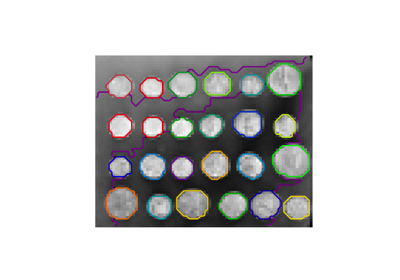A demo of structured Ward hierarchical clustering on an image of coins

A demo of structured Ward hierarchical clustering on an image of coinsAgglomerative clustering with and without structure

Agglomerative clustering with and without structure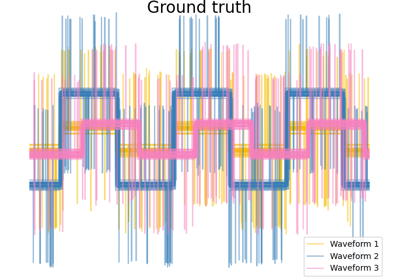Agglomerative clustering with different metrics

Agglomerative clustering with different metrics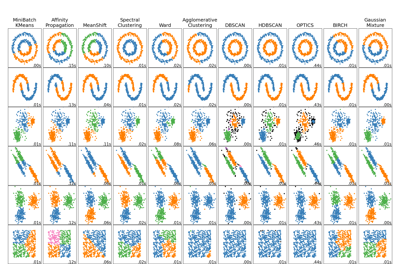Comparing different clustering algorithms on toy datasets

Comparing different clustering algorithms on toy datasets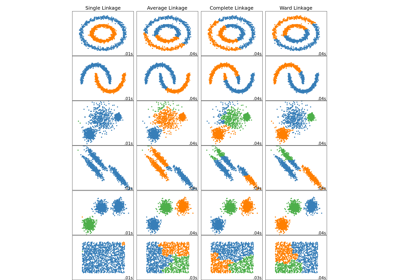Comparing different hierarchical linkage methods on toy datasets

Comparing different hierarchical linkage methods on toy datasets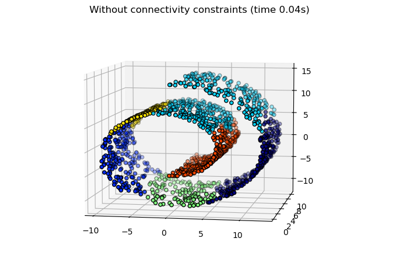Hierarchical clustering: structured vs unstructured ward

Hierarchical clustering: structured vs unstructured ward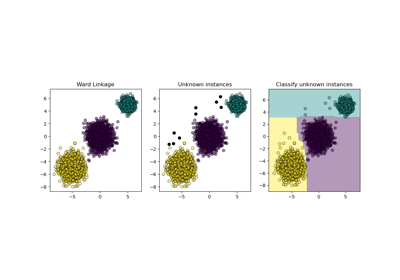Inductive Clustering

Inductive Clustering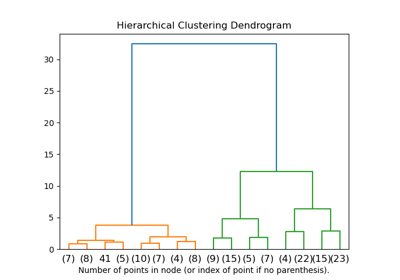Plot Hierarchical Clustering Dendrogram

Plot Hierarchical Clustering Dendrogram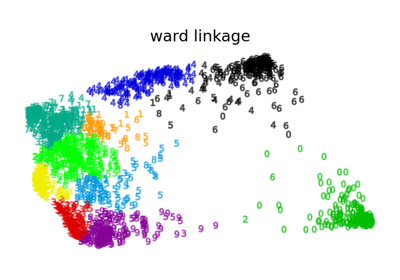Various Agglomerative Clustering on a 2D embedding of digits

Various Agglomerative Clustering on a 2D embedding of digits